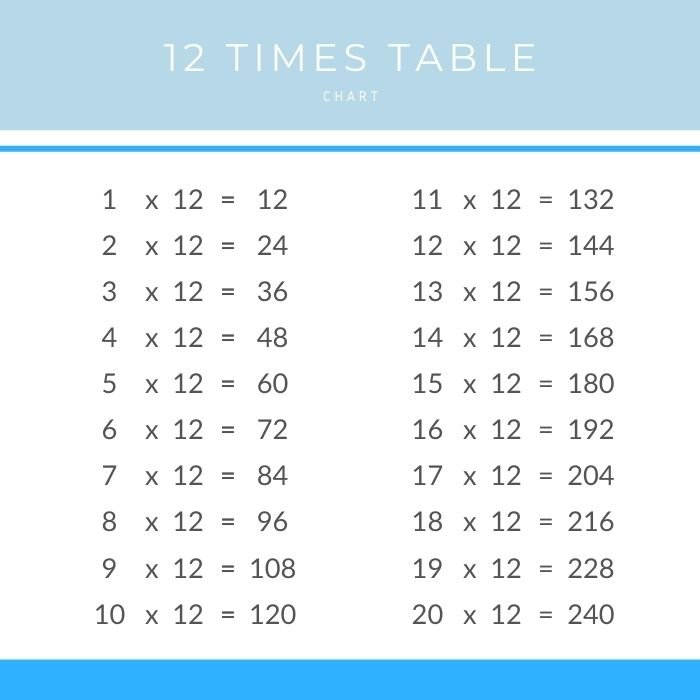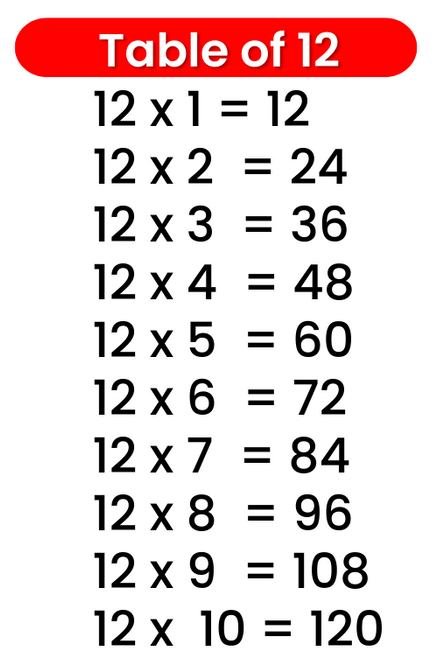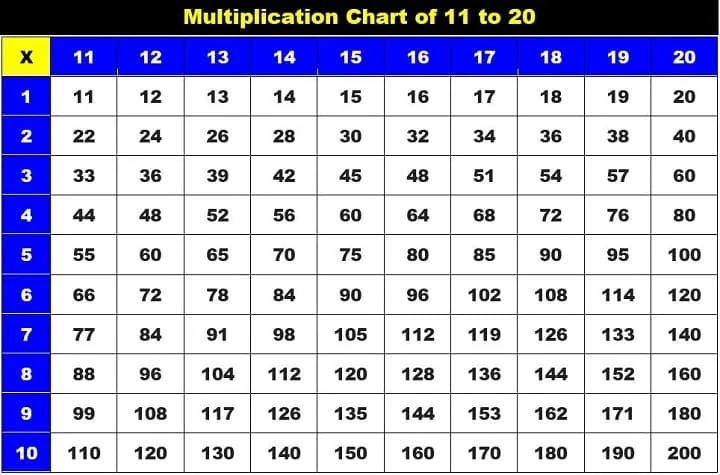# Times Table of 12, 12 ka table

## Times Table of 12, 12 ka table

Maths Multiplication Table of 12, Times Table of 12, 12 ka table, 12 ki Table Chart

12 ×‌ 1 = 12
12 ×‌ 2 = 24
12 ×‌ 3 = 36
12 ×‌ 4 = 48
12 ×‌ 5 = 60
12 ×‌ 6 = 72
12 ×‌ 7 = 84
12 ×‌ 8 = 96
12 ×‌ 9 = 108
12 ×‌ 10 = 120

## 12 ka table### Multiplication Tables of twelve upto 20### Times Table of 12 chart